# The power of origami

December 2009

### Doubling the cube using origami — proof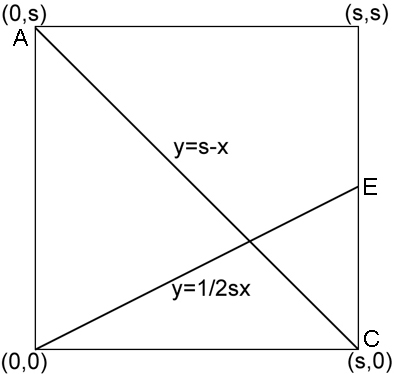Figure 1

First we show that the first three steps amount to dividing the height of the square into three equal parts. Place the square in a coordinate system with corner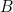at the point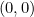. See figure 1. Now the line from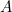to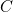has equation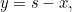whereis the side length of the square. The line fromto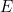has equation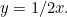So the two lines meet at a point with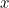-coordinate satisfying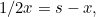so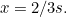The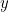-coordinate of the intersection point is therefore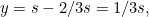as required.

The construction claims that the side length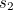of the new cube is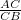times the side length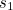of the given cube. Now since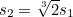, we have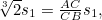so we need to show that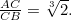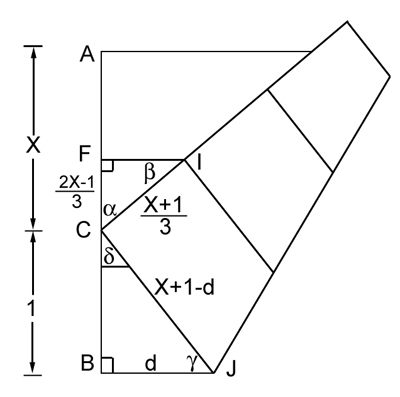Figure 2

Firstly, we can assume that the length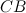is equal to 1, as we can scale the image as we like without affecting the proportions. We'll write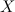for the length of the line segment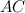, so the side length of our square is equal to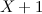. See figure 2. The line segment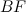corresponds to two thirds of a side of the square and therefore has length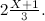This implies that the length of the line segment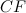is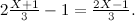We write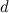for the length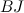. Since the line segment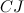is part of the bottom edge, its length is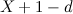. There is a right angle at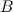, so by Pythagoras' theorem we have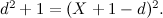This means that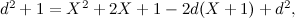and therefore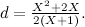Now consider the two right-angled triangles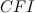and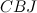. Considering the 180 degree angle formed at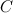by the vertical edge of the square, we get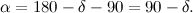The angles of the triangleadd up to 180 degrees, so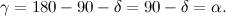So the trianglesandhave two equal angles: the right angle and the angle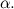This means that they are similar. Therefore, the ratios of corresponding sides of the two triagles are equal. Observing that the side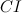of the triangleis a third of the side of the square and therefore has length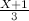, we get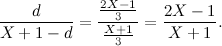Substituting the expression forabove gives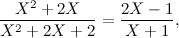so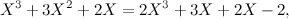so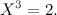This proves the result.